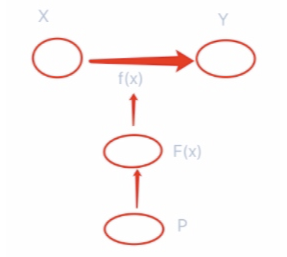# 梯度提升决策树公式推导

$\begin{equation}\begin{split} -\left[\frac {\partial L(y,f(x_i))}{\partial f(x_i)}\right]_{f(x)=f_{m-1}(x)} \end{split}\end{equation}$f的最终表示可以用w和b这种权重和偏置来表示。

$$\begin{equation}\begin{split} \frac {\partial f(w)}{\partial w}=0 \end{split}\end{equation}$$ 求得 $$w*$$
$$w*$$如何求得？
$$w^* =arg\min L(w)$$

$$F^w = arg\min L(f) \\ \frac {\partial L(f)}{\partial f}=0\\ f=f-\beta\frac {\partial L(f)}{\partial f} ,\beta=1$$

$\begin{equation}\begin{split} T(x;\Theta) = \sum_{j=1}^j c_jI(x\in R_j) \end{split}\end{equation}$

• 输入:训练数据集 $$T=\lbrace(x_1,y_1),(x_2,y_2),...,(x_N,y_N)\rbrace$$

• 输出：梯度提升决策树$$\hat f(x)$$
(1)初始化

$\begin{equation}\begin{split} f_0(x)=arg\min_c\sum_{i=1}^NL(y_i,c) \end{split}\end{equation}$

(2) 对 $$m=1,2,...,M$$

• (a) 对 $$i=1,2,...,N$$ 计算

$r_{mi} = -\left[\frac {\partial L(y,f(x_i))}{\partial f(x_i)}\right]_{f(x)=f_{m-1}(x)}$

• (b) 对$$r_mi$$拟合一个回归树，得到第m颗树的叶节点区域 $$R_{mj},j=1,2,...,J$$
• (c) 对 $$R_{mj},j=1,2,...,J$$，计算

$c_{mj}=arg\min_c\sum_{x\in R_{mj}}L(y_i,f_{m-1}(x_i)+c)$

这个地方也是将来xgboost要改进的点。经验风险最小化容易导致过拟合，可以采用正则化项。(xgboost第二个改进)

• (d) 更新$$f_m(x)=f_{m-1}(x)+\sum_{j=1}^J c_{mj}I(x \in R_{mj})$$

(3)得到回归梯度提升决策树

$\begin{equation}\begin{split} \hat f(x)=f_M(x)=\sum_{m=1}^M\sum_{j=1}^Jc_{mj}I(x \in R_{mj}) \end{split}\end{equation}$

posted @ 2020-12-18 15:17  ZzUuOo666  阅读(85)  评论(0编辑  收藏  举报